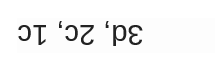SQL injection mini-quizAnswer the questions below to check your basic knowledge on SQL injection. There is always only 1 correct answer. (See the correct answers at the bottom of this page.)

If you need help you will find the answers in the 2nd video of the Mastering SQL injection course (Section 1).

1. Which one is NOT a valid comment sign in case of MySQL?
a) #
b) --
c) //
d) /*

2. Which option of sqlmap is required to test an application which expects the input with POST method?
a) --get
b) --post
c) --data
d) --send

3. Which one can be used to bypass a basic login screen in case of MySQL?
a) 'OR 1=1//
b) 'OR 1=1
c) 'OR '1'=1
d) 'OR 1=1-- -

------------------------------------------------------------------------------------------------------------------------